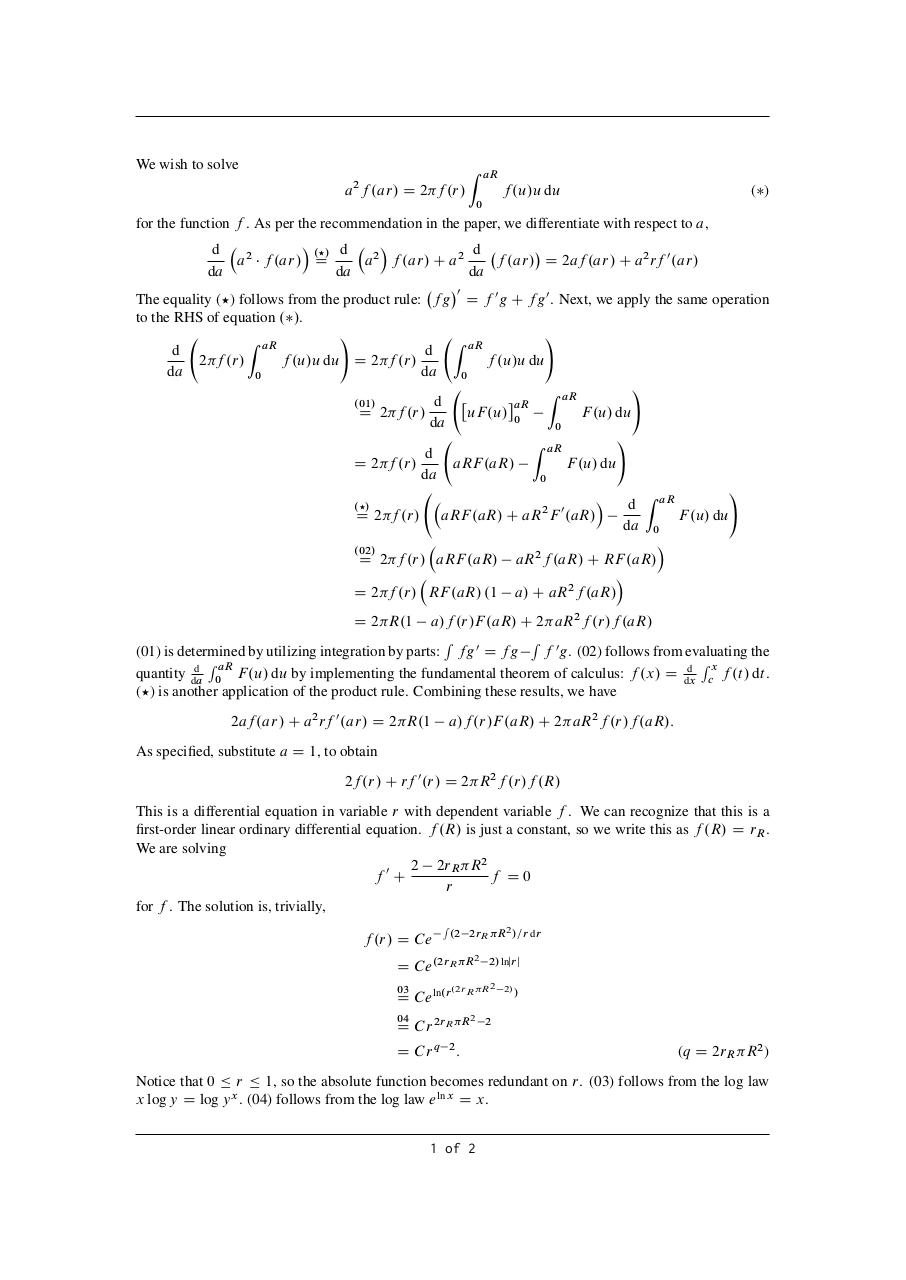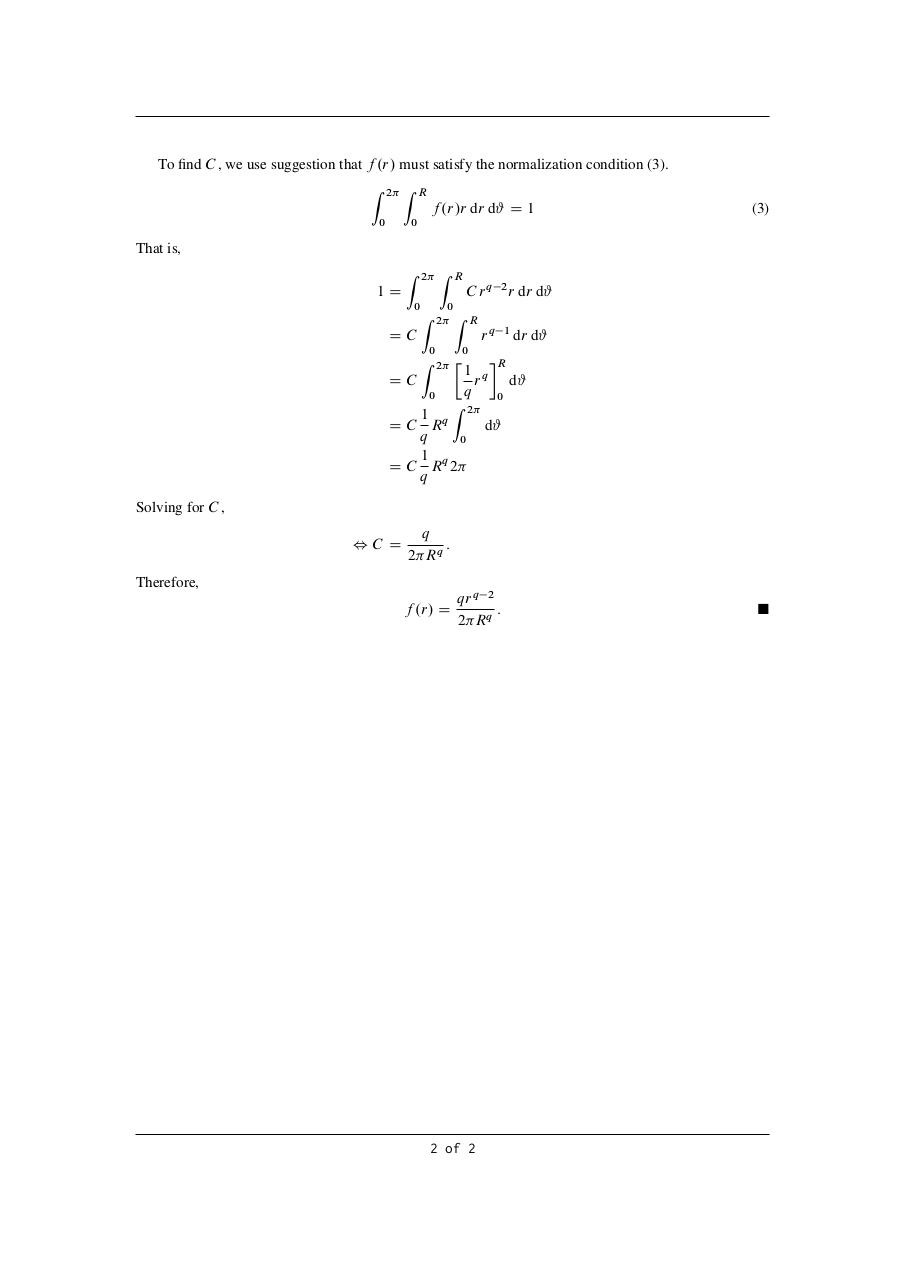# PDF Archive

Easily share your PDF documents with your contacts, on the Web and Social Networks.

## Temp .pdf

Original filename: Temp.pdf

This PDF 1.5 document has been generated by TeX / MiKTeX pdfTeX-1.40.17, and has been sent on pdf-archive.com on 17/04/2017 at 12:09, from IP address 49.177.x.x. The current document download page has been viewed 223 times.
File size: 106 KB (2 pages).
Privacy: public file### Document preview

We wish to solve

aR

Z

2

a f .ar/ D 2 f .r/

f .u/u du

( )

0

for the function f . As per the recommendation in the paper, we differentiate with respect to a,
.?/ d

d
d 2
a f .ar/ D
a2 f .ar/ C a2
f .ar/ D 2af .ar/ C a2 rf 0 .ar/
da
da
da
0
The equality (?) follows from the product rule: fg D f 0 g C fg 0 . Next, we apply the same operation
to the RHS of equation . /.
!
!
Z aR
Z aR
d
d
2 f .r/
f .u/u du D 2 f .r/
f .u/u du
da
da
0
0
!
Z aR
aR
d
.01/
D 2 f .r/
uF .u/ 0
F .u/ du
da
0
!
Z aR
d
D 2 f .r/
aRF .aR/
F .u/ du
da
0
!
Z aR

d
.?/
2 0
F .u/ du
D 2 f .r/ aRF .aR/ C aR F .aR/
da 0

.02/
D 2 f .r/ aRF .aR/ aR2 f .aR/ C RF .aR/

D 2 f .r/ RF .aR/ .1 a/ C aR2 f .aR/
a/f .r/F .aR/ C 2 aR2 f .r/f .aR/
R
R 0
(01) is determined by utilizing integration by parts: fg 0 D fg
f g. (02) follows from evaluating the
R aR
Rx
d
d
quantity da 0 F .u/ du by implementing the fundamental theorem of calculus: f .x/ D dx
c f .t / dt .
(?) is another application of the product rule. Combining these results, we have
D 2 R.1

2af .ar/ C a2 rf 0 .ar/ D 2 R.1

a/f .r/F .aR/ C 2 aR2 f .r/f .aR/:

As specified, substitute a D 1, to obtain
2f .r/ C rf 0 .r/ D 2 R2 f .r/f .R/
This is a differential equation in variable r with dependent variable f . We can recognize that this is a
first-order linear ordinary differential equation. f .R/ is just a constant, so we write this as f .R/ D rR .
We are solving
2 2rR R2
f0C
f D0
r
for f . The solution is, trivially,
f .r/ D C e

R

.2 2rR R2 /=r dr

D C e .2rR R

2

2/ lnjrj

03

.2rR R2

04

2

D C e ln.r

D C r 2rR R
D C rq

2

:

2/ /

2

(q D 2rR R2 )

Notice that 0 r 1, so the absolute function becomes redundant on r. (03) follows from the log law
x log y D log y x . (04) follows from the log law e ln x D x.
1 of 2

To find C , we use suggestion that f .r/ must satisfy the normalization condition (3).
Z

2

R

Z

f .r/r dr d# D 1
0

(3)

0

That is,
Z

2

Z

R

Z

0
2 Z R

C rq

1D
0

DC
0

rq

r dr d#

1

dr d#

0
2

Z

2

DC
0

1 q
r
q

R
d#
0

Z 2
1
d#
D C Rq
q
0
1
D C Rq 2
q
Solving for C ,
,C D

q
:
2 Rq

Therefore,
f .r/ D

qr q 2
:
2 Rq

2 of 2